# Valuation method

ref: Investopedia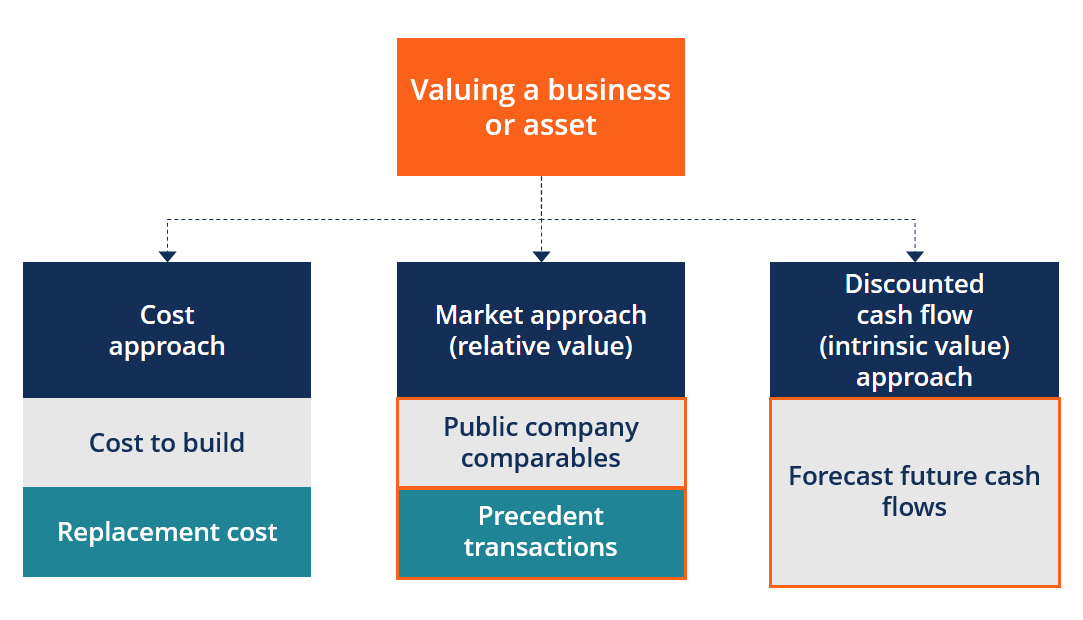2 categories of valuation models

• absolute
• attempt to find the intrinsic or "true" value of an investment based only on fundamentals, such things as dividends, cash flow, and the growth rate for a single company and not worry about any other companies
• notable method: Discounted Cash Flow model (DCF) and its variant Dividend Discount Model (DDM)
• relative
• compare the company in question to other similar companies. These methods involve calculating multiples and ratios, such as the price-to-earnings (P/E) ratio, and comparing them to the multiples of similar companies## Comparables Model using Financial Ratios

ref: Investopedia

The comparable analysis is an example of relative stock valuation. It compares the stock's price multiples to a benchmark to determine if the stock is relatively undervalued or overvalued.

ref:

Financial ratios are powerful tools to help summarize financial statements and the health of a company.

Financial ratios are typically cast into four categories:

• Profitability ratios
• Liquidity ratios
• Solvency ratios
• Valuation ratios or Multiples

## Profitability Ratios

Profitability ratios assess a company's ability to earn profits from its sales or operations, balance sheet assets, or shareholders' equity.

### Key profitability ratios

• Gross margin (and adjusted gross margin)
• Operating margin
• Net profit margin
• EBITDA margin
• Operating cash flow margin
• Return on assets (ROA)
• Return on Equity (ROE)
• Return on invested capital (ROIC)
• Return on investment (ROI)

### Understanding profitability ratios

Higher ratio results are often more favorable, but these ratios provide much more information when compared to results of similar companies, the company's own historical performance, or the industry average.

Profitability ratios indicate how efficiently a company generates profit and value for shareholders.

## Liquidity Ratios

Liquidity measures how quickly a company can repay its debts. It also shows how well company assets cover expenses.

### Understanding liquidity ratios

Liquidity ratios give investors an idea of a company’s operational efficiency. They also show how quickly and easily a company generates cash to purchase additional assets or to repay creditors quickly, either in an emergency situation or in the course of normal business.

## Solvency Ratios

Solvency ratios, also known as leverage ratios, are used by investors to get a picture of how well a company can deal with its long-term financial obligations.

• Debt to total assets
• Debt-to-Equity (D/E) ratio
• Time interest earned
• Interest coverage ratio
• Net income to liabilities
• Times interest earned

## Valuation Ratios aka Multiples

Valuation ratios, also known as Multiples, are some of the most commonly quoted and easily used ratios for analyzing the attractiveness of an investment in a company.

A multiple measures some aspect of a company's financial well-being, determined by dividing one metric by another metric.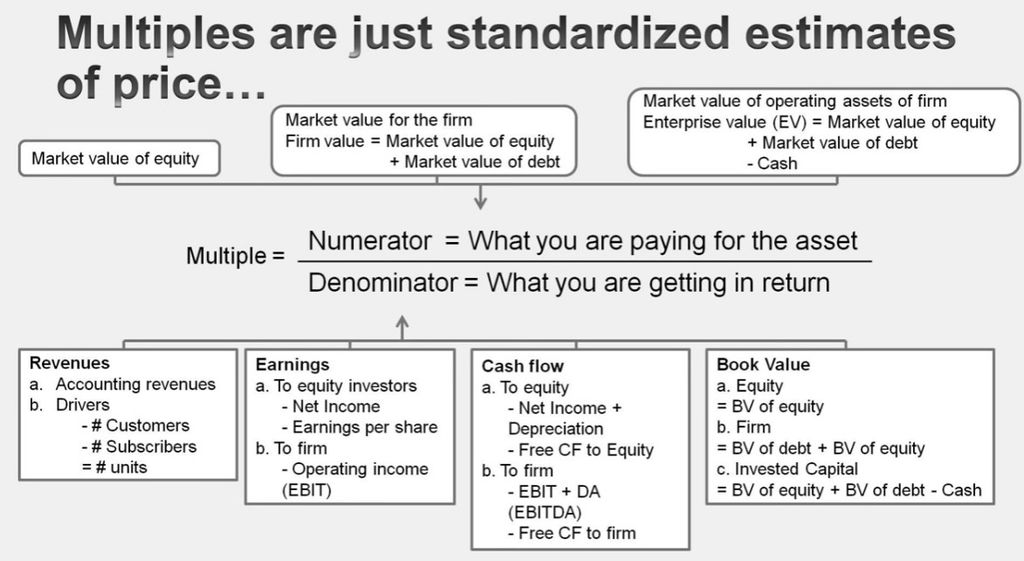## Dividend Discount Model

ref: Investopedia

The dividend discount model (DDM) is a quantitative method used for predicting the price of a company's stock based on the theory that its present-day price is worth the sum of all of its future dividend payments when discounted back to their present value.

The DDM is based on the theory that the value of a company is the present worth of the sum of all of its future dividend payments.

DDM is a variant of DCF model, called an equity discounted cash flow model.

## Formula

Mathetically,

$Value\ of\ Stock = \frac{EDPS}{CCE-DGR}$

where

• $EDPS =$ Expected Dividend per Share
• $CCE =$ Cost of Capital Equity
• $DGR =$ Dividend Growth Rate

### Gordon growth model (GGM)

The most common and straightforward variant of the Dividend Discount Model (DDM) is known as the Gordon growth model (GGM)

The GGM assumes that a company exists forever and that there is a constant growth in dividends when valuing a company's stock.

$P = \frac{D_0*(1+g)}{r-g} = \frac{D_1}{r-g}$

where

• $P =$ current stock Price
• $D_0 =$ the most recent dividend
• $D_1 =$ value of next year's dividend (after $D_0$)
• $r =$ constant Cost of Capital Equity for the company, or rate of return
• $g =$ constant Growth rate expected for dividends, in perpetuity

## Shortcomings of the DDM

• The model assumes a constant dividend growth rate in perpetuity
• DDM may not be the best model to value newer companies that have fluctuating dividend growth rates or no dividend at all
• The second issue with the DDM is that the output is very sensitive to the inputs
• The model also fails when companies may have a lower rate of return (r) compared to the dividend growth rate (g). This may happen when a company continues to pay dividends even if it is incurring a loss or relatively lower earnings

## Discounted Cash Flow Model

ref:

Discounted cash flow (DCF) analysis attempts to figure out the value of an investment today, based on projections of how much money it will generate in the future.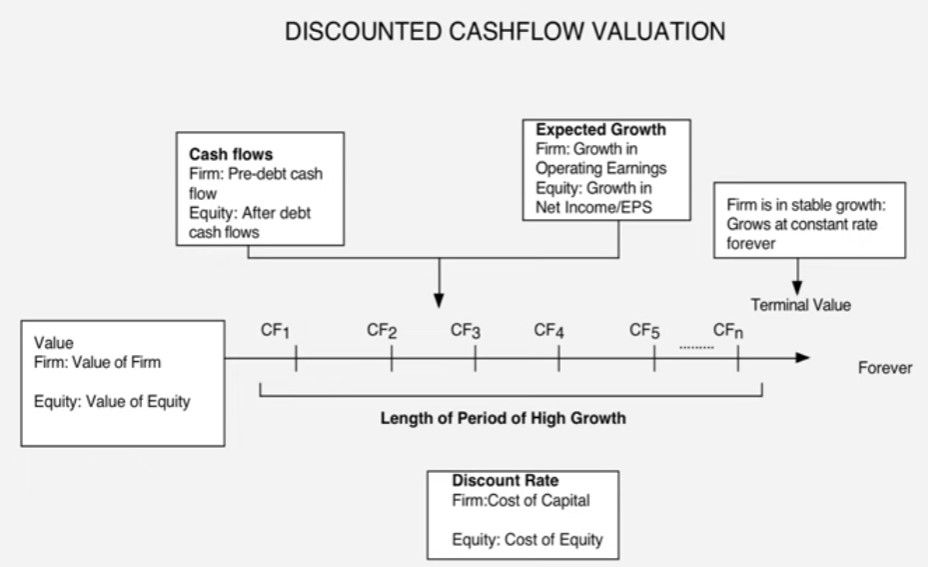## Formula

In general,

$Value = \frac{CF_1}{(1+r)^1} + \frac{CF_2}{(1+r)^2} + ... + \frac{CF_n}{(1+r)^n}$

where

• $CF =$ the cash flow for the given year
• $CF_1 =$ the cash flow for the 1st year
• $CF_2 =$ the cash flow for the 2nd year
• $CF_n =$ the cash flow for the year $n^{th}$
• $r =$ the discount rate
• Companies typically use the weighted average cost of capital (WACC) for the discount rate, because it takes into consideration the rate of return expected by shareholders

## What DCF Can Tell You

The purpose of DCF analysis is to estimate the money an investor would receive from an investment, adjusted for the time value of money. The time value of money assumes that a dollar today is worth more than a dollar tomorrow because it can be invested.

## Variations of DCF

### Which DCF model to choose?

The fundamental choices for DCF valuation:

• Cashflows to Discount
• Dividends
• for firms which pay dividends (and repurchase stock) which are close to the Free Cash Flow to Equity (over a extended period)
• for firms where FCFE are difficult to estimate (Example: Banks and Financial Service companies)
• Free Cash Flows to Equity (FCFE)
• for firms which pay dividends which are significantly higher or lower than the Free Cash Flow to Equity. (What is significant? ... As a rule of thumb, if dividends are less than 75% of FCFE or dividends are greater than FCFE)
• for firms where dividends are not available (Example: Private Companies, IPOs)
• for firms which have stable leverage, whether high or not, and
• if equity (stock) is being valued
• Free Cash Flows to Firm (FCFF)
• for firms which have high leverage, and expect to lower the leverage over time, because
• debt payments do not have to be factored in
• the discount rate (cost of capital) does not change dramatically over time.
• for firms for which you have partial information on leverage (eg: interest expenses are missing..)
• in all other cases, where you are more interested in valuing the firm than the equity.
• Expected Growth. Use the growth model only if cash flows are positive
• Stable Growth
• Two Stages of Growth: High Growth -> Stable Growth
• Use the two-stage growth model if the firm is growing at a moderate rate ( within 8% of the stable growth rate)
• Three Stages of Growth: High Growth -> Transition Period -> Stable Growth
• Use the three-stage growth model if the firm is growing at a high rate ( more than 8% higher than the stable growth rate)
• Discount Rate: should be consistent with the cash flow being discounted
• Cash Flow to Equity -> Cost of Equity
• Cash Flow to Firm -> Cost of Capital
• Base Year Numbers
• Current Earnings / Cash Flows
• Normalized Earnings / Cash Flows

Fig: The building blocks of Valuation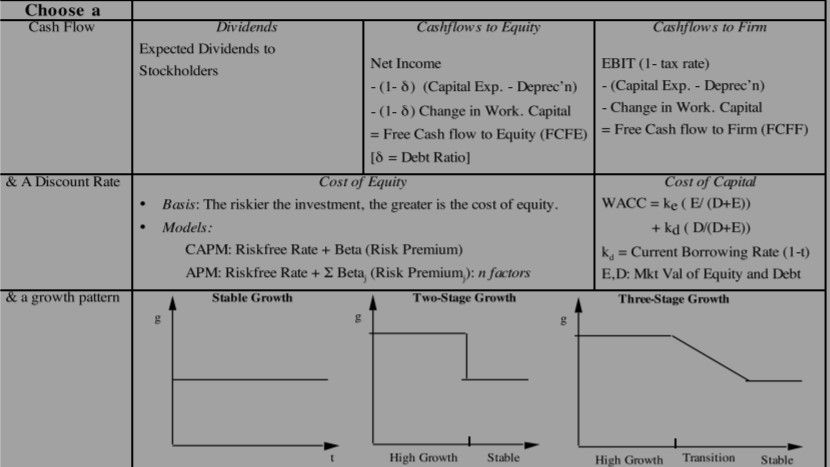#### Three stages of Growth

According to Sharpe, Alexander, and Bailey 1999 1, growth falls into three stages:

• Growth phase
• A company in its growth phase typically enjoys rapidly expanding markets, high profit margins, and an abnormally high growth rate in earnings per share
• Companies in this phase often have negative free cash flow to equity because the company invests heavily in expanding operations
• Given high prospective returns on equity, the dividend payout ratios of growth-phase companies are often low or even zero
• As the company’s markets mature or as unusual growth opportunities attract competitors, earnings growth rates eventually decline
• Transition phase
• In this phase, which is a transition to maturity, earnings growth slows as competition puts pressure on prices and profit margins or as sales growth slows because of market saturation
• earnings growth rates may be above average but declining toward the growth rate for the overall economy
• Capital requirements typically decline in this phase, often resulting in positive free cash flow and increasing dividend payout ratios (or the initiation of dividends)
• Mature phase
• In maturity, the company reaches an equilibrium in which investment opportunities on average just earn their opportunity cost of capital
• The dividend and earnings growth rate of this phase is called the mature growth rate
• This phase reflects the stage in which a company can properly be valued using the Gordon growth model

Fig: Three typical growth patterns## Growth and Firm Characteristics

Dividend Discount ModelFCFE Discount ModelFCFF Discount Model
High growth firms generally- Pay no or low dividends
- Earn high returns on projects (ROA)
Have low leverage (D/E)
- Have high risk (high betas)
- Have high capital expenditures relative to depreciation.
- Earn high returns on projects
- Have low leverage
- Have high risk
- Have high capital expenditures relative to depreciation
- Earn high returns on projects
- Have low leverage
- Have high risk
Stable growth firms generally- Pay large dividends relative to earnings (high payout)
- Earn moderate returns on projects (ROA is closer to market or industry average)
- Have higher leverage
- Have average risk (betas are closer to one)
- narrow the difference between cap ex and depreciation (Sometimes they offset each other)
- Earn moderate returns on projects (ROA is closer to market or industry average)
- Have higher leverage
- Have average risk (betas are closer to one)
- narrow the difference between cap ex and depreciation (Sometimes they offset each other)
- Earn moderate returns on projects (ROA is closer to market or industry average)
- Have higher leverage
- Have average risk (betas are closer to one)

## Footnotes

1. Sharpe, W. F., Alexander, G. J., & Bailey, J. V. (1999). Investments: International Edition (6th ed.). London: Prentice Hall International Inc. URL to Google Books˄

#### Summary - DCF model choice

Fig: Steps to choose DCF model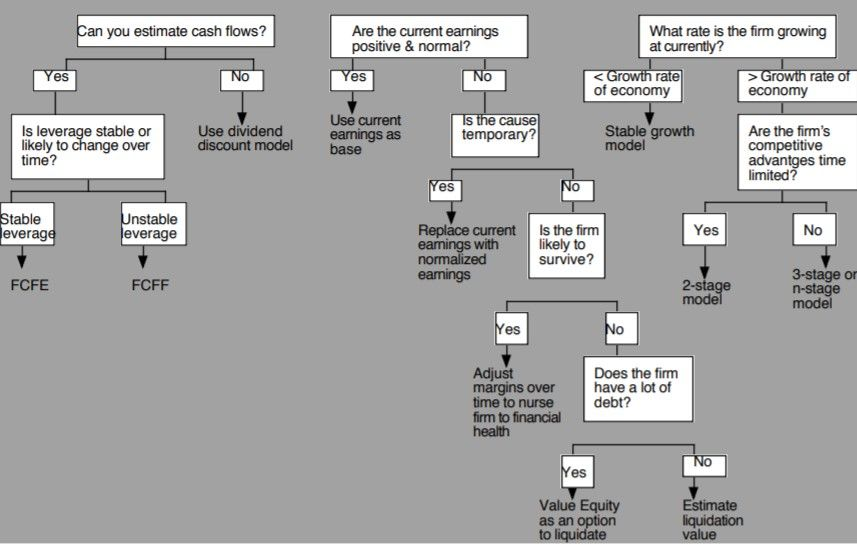Dividend Discount ModelFCFE ModelFCFF Model
Stable Growth Model- Growth rate in firm's earnings is stable ($g_{firm-economy}$+1%)
- Dividends are close to FCFE (or) FCFE is difficult to compute
- Leverage is stable
- Growth rate in firm's earnings is stable ($g_{firm-economy}$+1%)
- Dividends are very different from FCFE (or) Dividends not available (Private firm)
- Leverage is stable
- Growth rate in firm's earnings is stable (\$g_{firm-economy}+1%)
- Leverage is high and expected to change over time (unstable)
Two-Stage Model- Growth rate in firm's earnings is moderate
- Dividends are close to FCFE (or) FCFE is difficult to compute
- Leverage is stable
- Growth rate in firm's earnings is moderate
- Dividends are very different from FCFE (or) Dividends not available (Private firm)
- Leverage is stable
- Growth rate in firm's earnings is moderate
- Leverage is high and expected to change over time (unstable)
Three-Stage Model- Growth rate in firm's earnings is high
- Dividends are close to FCFE (or) FCFE is difficult to compute
- Leverage is stable
- Growth rate in firm's earnings is high
- Dividends are very different from FCFE (or) Dividends not available (Private firm)
- Leverage is stable
- Growth rate in firm's earnings is high
- Leverage is high and expected to change over time (unstable)

### DCF model for Firm with stable growth

aka Gordon growth model or constant growth forever

$V_0=\frac{CF_1}{r-g}$

where:

• $V_0=$ Value of Equity (if cash flows to equity are discounted) or Firm (if cash flows to firm are discounted)
• $CF_1=$ Cash Flow in period after the most recent $CF_0$; Dividends or FCFE if valuing equity; FCFF if valuing firm
• $r=$ Cost of Equity (if discounting Dividends or FCFE) or Cost of Capital (if discounting FCFF)
• $g=$ Expected growth in Cash Flow being discounted in stable period

Use the stable growth model, if the firm is growing at a rate which is below or close (within 1-2%) to the growth rate of the economy

• pp. v3_440 - v3_448. Section 3 The Gordon Growth Model. Book 2022 CFA Program Curriculum Level II Box Set Volumes 1-6. Calibre Library id:"=1828".

### DCF model for Firm with two distinct stages of growth

aka two-stage growth model.

Basically, the two-stage DCF is a multiple-period model. It means that simply find the present value of the first $n$ dividends and the present value of the projected value at time $n$

$V_0 = \displaystyle\sum_{t=1}^n \frac{CF_t}{(1+r)^t} + \frac{V_n}{(1+r)^n}$

The two-stage model assumes that the first $n$ dividends grow at an extraordinary short-term rate, $g_S$.

$CF_t = CF_0(1+g_S)^t$

After time $n$, the annual dividend growth rate changes to a normal long-term rate, $g_L$. The cash flow at time $n+1$ is:

$CF_{n+1} = CF_n(1+g_L) = CF_0(1+g_S)^n(1+g_L)$

Applying Gordon growth model to find:

$V_n = \frac{CF_{n+1}}{r-g_L} = \frac{CF_0(1+g_S)^n(1+g_L)}{r-g_L}$

Which leads to the formula to find the value at $t=0$

$V_0 = \displaystyle\sum_{t=1}^n \frac{CF_0(1+g_S)^t}{(1+r)^t} + \frac{CF_0(1+g_S)^n(1+g_L)}{(1+r)^n(r-g_L)}$

where:

• $V_0=$ Value of Equity (if cash flows to equity are discounted) or Firm (if cash flows to firm are discounted)
• $CF=$ Cash Flow in period t; Dividends or FCFE if valuing equity; FCFF if valuing firm
• $r=$ Cost of Equity (if discounting Dividends or FCFE) or Cost of Capital (if discounting FCFF)
• $g_S=$ Expected high growth short-term rate for the initial period
• $g_L=$ Expected sustainable growth long-term rate for the next period

Suitable for companies that are not expected to grow at a constant rate over time. These companies tend to be high-growth initially and become stable after a couple of years.

• pp. v3_456 - v3_461. Section 6.1 Multistage Dividend Discount Models: Two-Stage Dividend Discount Model and Valuing a non-dividend paying company. Book 2022 CFA Program Curriculum Level II Box Set Volumes 1-6. Calibre Library id:"=1828".

### DCF model for Firm with three distinct stages of growth

aka three-stage growth model.

#### H-model

Fuller and Hsia (1984) developed H-model, a variant of the two- stage model in which growth begins at a high rate and declines linearly throughout the supernormal growth period until it reaches a normal rate at the end.

The value of the dividend stream in H-model is the combination of

• the present value of the company’s dividend stream if it were to grow at $g_L$ forever
• and an approximation of the extra value (assuming $g_S > g_L$) accruing to the stock because of its supernormal growth for Years $1$ through $2H$

$V_0 = \frac{D_0(1+g_L)}{r-g_L} + \frac{D_0H(g_S-g_L)}{r-g_L}$

where:

• $V_0=$ value per share at $t=0$
• $D_0=$ current dividend
• $r=$ required rate of return on equity
• $H=$ half-life in years of the high-growth period (i.e., high-growth period $=2H$)
• $g_S=$ initial short-term dividend growth rate
• $g_L=$ normal long-term dividend growth rate after year $2H$

#### Three-Stage Model

Two popular versions, distinguished by the modeling of the second stage

• the company is assumed to have three distinct stages of growth and the growth rate of the second stage is typically constant
• In the second version, the growth rate in the middle (second) stage is assumed to decline linearly to the mature growth rate: essentially, the second and third stages are treated as an H-model

• pp. v3_461 - v3_461. Section 7 The H-model and three-stage Dividend Discount Model. Book 2022 CFA Program Curriculum Level II Box Set Volumes 1-6. Calibre Library id:"=1828".

### Excess Returns Model

ref: SimplyWallSt

Used for financial companies such as banks and insurance firms. These companies generally do not have a significant proportion of physical assets and face different regulatory requirements for cash holdings to other businesses.

Excess Returns method is better suited to calculate the intrinsic value of financial companies than the traditional discounted cash flows model. The key assumption for this model is that equity value is how much the firm can earn, over and above its cost of equity, given the level of equity it has in the company at the moment. The returns above the cost of equity is known as excess returns:

Excess Return = (Return on Equity – Cost Of Equity) \ (Book Value Of Equity)

We use this value to calculate the terminal value of the company, which is how much we expect the company to continue to earn every year, forever. This is a common component of discounted cash flow models:

$Terminal Value = \frac{Excess Return}{Cost of Equity – Expected Growth Rate}$

Putting this all together, we get the value of the company:

Company Valuation = Book Value of Equity + Present Value of Terminal Value

$Value Per Share = \frac{Book Value of Equity + Present Value of Terminal Value}{Shares Outstanding}$

Note that the component Book Value of Equity (BVE) represents the equity capital that has been invested in the company plus retained earnings. For each year going forward, BVE is estimated in order to reflect the growing nature of the company.

### Adjusted-Funds-From-Operations (AFFO) 2-Stage Discounted Cash Flow Model

ref: SimplyWallSt

Used for Real Estate Investment Trusts (REITs) as these companies incur capital gains and other real estate-specific factors which impacts their free cash flows.

This model is the same as the 2-Stage Discounted Cash Flows Model, except for one key difference – instead of discounting the company's free cash flows, we use its Adjusted Funds from Operations (AFFO) instead. AFFO better reflects the operational cash flows of REITs as opposed to the commonly used free cash flows.

Funds from Operations (FFO) is the company's earnings, with depreciation and amortization added back on, and removal of capital gains from property sales. AFFO has other adjustments the FFO number to make it even more accurate, by subtracting off capital expenditure and maintenance costs of the property, and adding rental increases. These factors are very specific to property and REITs, making it a far superior measure of value for these types of stocks.

### Dividend Discount Model (DDM)

Suitable for companies that consistently pay out a meaningful portion of their earnings as dividends.

Children